# ProblemsThree point charges, q1 = - 4 nC, q2 = 5 nC, and q3 = 3 nC, are placed as in Fig. 1.4.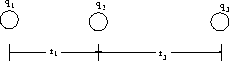If r1 = 0.5 m and r3 = 0.8 m, find the force on q2 due to the other two charges.

Solution:
We first find the force on q2 due to q1 :

F1 = k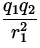= 9.0 x 109 x= 7.2 x 10- 7 N ,

which is directed to the left. The force on q2 due to q3 is found as:

F3 = k= 9.0 x 109 x= 2.11 x 10- 7 N ,

which is also directed to the left. Thus, the total force on q2 is given by

7.2 x 10- 7 + 2.11 x 10- 7 = 9.31 x 10- 7 N .

Thus, the total force on q2 is 9.31 x 10- 7 N directed to the left.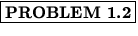The charges in Fig. 1.5, with q1 = q = - q2 , form what is called a dipole. Find the electric field a distance d along the x -axis.Solution:
We resolve the contributions from q1 and q2 into components. For q1 ,

 (E1)x = k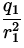cos= k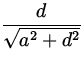, (E1)y = - ksin= - k,
while for q2 ,
 (E2)x = - k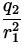cos= - k, (E2)y = - ksin= k.
The total electric field then has components
 Ex = (E1)x + (E2)x = 0, Ey = (E1)y + (E2)y = - 2k.
Thus, the net electric field is directed in the - y direction and has a magnitude of - 2kqa/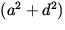3/2.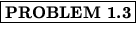A m = 2 g ball is suspended by a l = 20 cm long string as in Fig. 1.6 in a constant electric field of E = 1000 N/C. If the string makes an angle of= 15 o with respect to the vertical, what is the net charge on the ball?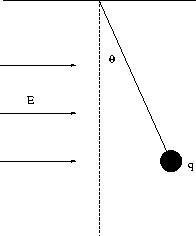Solution:
We first examine in Fig. 1.7 the forces present on the ball.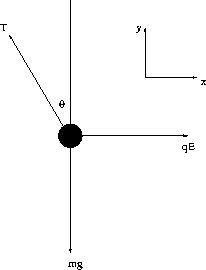In equilibrium the net force on the ball is zero; we thus have, in the y direction,

Tcos- mg = 0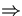T =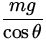,

and in the x direction,

qE - Tsin= 0q =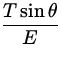=.

With the numbers given, we find

q =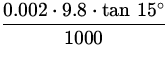= 5.2 x 10- 6 C .

Thus, the charge on the ball is 5.2C.The three charges in Fig. 1.8, with q1 = 8 nC, q2 = 2 nC, and q3 = - 4 nC, are separated by distances r2 = 3 cm and r3 = 4 cm. How much work is required to move q1 to infinity?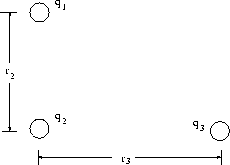Solution:
We will first calculate the electric potential V due to a point charge q a distance r away as

V = k,

which assumes the potential vanishes at infinity. The potential due to q2 at the point occupied by q1 is thus

V2 = k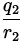= 9.0 x 109 x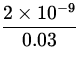= 600 V ,

while that due to q3 is

V3 = k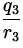= 9.0 x 109 x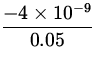= - 720 V ,

The net potential is thus

V = 600 + (- 720) = - 120 V ,

and so the work required to move q1 to infinity is

W = - q1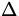V = - q1 x (Vf - Vi) = - 8 x 10- 9 x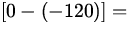- 9.6 x 10- 7 J .

Thus, the work required is 9.6 x 10- 7 J, with the minus sign indicating that a net work must be supplied.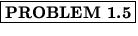A constant electric field E = 2000 V/m exists as in Fig. 1.9. A 10C charge of mass 20 g, initially at rest at x = - 1 m, is released. What is its speed at x = 5 m?Solution:
We first find the potential difference between the two points of interest:

E = -= -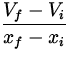V = - Ex = - 2000- 12,000 V .

The work done by the electric field in moving the charge is

W = - qV = - 10 x 10- 6(- 12,000) = 0.12 J .

The positive sign indicates the electric field is doing the work. This work goes into changing the kinetic energy of the particle:

W =K = Kf - Ki =mv 2 - 0v ==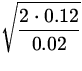= 3.46 m / s .

Thus, the final speed of the particle is 3.46 m/s.